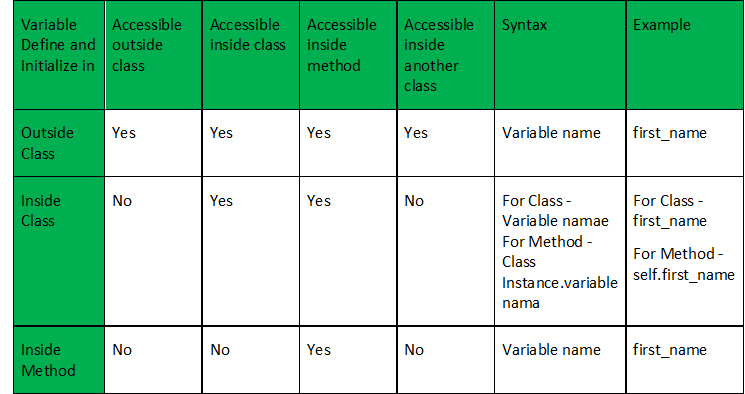# Python | Using variable outside and inside the class and method

• Last Updated : 18 May, 2020

In Python, we can define the variable outside the class, inside the class, and even inside the methods. Let’s see, how to use and access these variables throughout the program.

Variable defined outside the class:

The variables that are defined outside the class can be accessed by any class or any methods in the class by just writing the variable name.

 `# Program to demonstrate 'Variable  ` `# defined outside the class' ` ` `  `# Variable defined outside the class. ` `outVar ``=` `'outside_class'`     `print``(``"Outside_class1"``, outVar) ` ` `  `''' Class one '''` `class` `Geek: ` `    ``print``(``"Outside_class2"``, outVar) ` ` `  `    ``def` `access_method(``self``): ` `        ``print``(``"Outside_class3"``, outVar) ` ` `  `# Calling method by creating object ` `uac ``=` `Geek() ` `uac.access_method() ` ` `  `''' Class two '''` `class` `Another_Geek_class: ` `    ``print``(``"Outside_class4"``, outVar)  ` ` `  `    ``def` `another_access_method(``self``): ` `        ``print``(``"Outside_class5"``, outVar) ` ` `  `# Calling method by creating object ` `uaac ``=` `Another_Geek_class() ` `uaac.another_access_method() `

Output:

```Outside_class1 outside_class
Outside_class2 outside_class
Outside_class3 outside_class
Outside_class4 outside_class
Outside_class5 outside_class
```

Variable defined inside the class:

The variables that are defined inside the class but outside the method can be accessed within the class(all methods included) using the instance of a class. For Example – self.var_name.
If you want to use that variable even outside the class, you must declared that variable as a global. Then the variable can be accessed using its name inside and outside the class and not using the instance of the class.

 `# Program to demonstrate 'Variable  ` `# defined inside the class' ` ` `  `# print("Inside_class1", inVar) # Error ` ` `  `''' Class one'''` `class` `Geek: ` ` `  `    ``# Variable defined inside the class. ` `    ``inVar ``=` `'inside_class'` `    ``print``(``"Inside_class2"``, inVar) ` ` `  `    ``def` `access_method(``self``): ` `        ``print``(``"Inside_class3"``, ``self``.inVar) ` ` `  `uac ``=` `Geek() ` `uac.access_method() ` ` `  `''' Class two '''` `class` `another_Geek_class: ` `    ``print``() ` `# print("Inside_class4", inVar) # Error ` ` `  `    ``def` `another_access_method(``self``): ` `        ``print``() ` `# print("Inside_class5", inVar) # Error ` ` `  `uaac ``=` `another_Geek_class() ` `uaac.another_access_method() `

Output:

```Inside_class2 inside_class
Inside_class3 inside_class
```

The statements which are marked as error will produce an error upon execution as the variable is not accessible there.

Variable defined inside the method:

The variables that are defined inside the methods can be accessed within that method only by simply using the variable name. Example – var_name.
If you want to use that variable outside the method or class, you have to declared that variable as a global.

 `# Program to demonstrate 'Variable  ` `# defined inside the method' ` ` `  `# print("Inside_method1", inVar) # Error ` ` `  `'''class one'''` `class` `Geek: ` `    ``print``() ` `# print("Inside_method2", inVar) # Error ` ` `  `    ``def` `access_method(``self``): ` ` `  `        ``# Variable defined inside the method. ` `        ``inVar ``=` `'inside_method'` `        ``print``(``"Inside_method3"``, inVar) ` ` `  `uac ``=` `Geek() ` `uac.access_method() ` ` `  `'''class two'''` `class` `AnotherGeek: ` `    ``print``() ` `# print("Inside_method4", inVar) # Error ` ` `  `    ``def` `access_method(``self``): ` `        ``print``() ` `# print("Inside_method5", inVar) # Error ` ` `  `uaac ``=` `AnotherGeek() ` `uaac.access_method() `

Output:

```Inside_method3 inside_method
```

The statements which are marked as error will produce error upon execution as the variable is not accessible there.

Summary:My Personal Notes arrow_drop_up
Recommended Articles
Page :